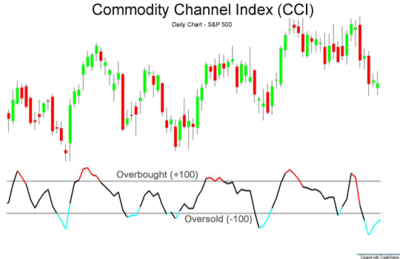# Commodity channel index

The commodity channel index (CCI) is an oscillator originally introduced by Donald Lambert in 1980.

Since its introduction, the indicator has grown in popularity and is now a very common tool for traders in identifying cyclical trends not only in commodities, but also equities and currencies. The CCI can be adjusted to the timeframe of the market traded on by changing the averaging period.

## Calculation

CCI measures a security’s variation from the statistical mean.

The CCI is calculated as the difference between the typical price of a commodity and its simple moving average, divided by the mean absolute deviation of the typical price. The index is usually scaled by an inverse factor of 0.015 to provide more readable numbers:,

where the pt is the, SMA is the simple moving average, and σ is the mean absolute deviation.

For scaling purposes, Lambert set the constant at 0.015 to ensure that approximately 70 to 80 percent of CCI values would fall between −100 and +100. The CCI fluctuates above and below zero. The percentage of CCI values that fall between +100 and −100 will depend on the number of periods used. A shorter CCI will be more volatile with a smaller percentage of values between +100 and −100. Conversely, the more periods used to calculate the CCI, the higher the percentage of values between +100 and −100.

## InterpretationTraders and investors use the commodity channel index to help identify price reversals, price extremes and trend strength. As with most indicators, the CCI should be used in conjunction with other aspects of technical analysis. CCI fits into the momentum category of oscillators. In addition to momentum, volume indicators and the price chart may also influence a technical assessment. It is often used for detecting divergences from price trends as an overbought/oversold indicator, and to draw patterns on it and trade according to those patterns. In this respect, it is similar to bollinger bands, but is presented as an indicator rather than as overbought/oversold levels.

The CCI typically oscillates above and below a zero line. Normal oscillations will occur within the range of +100 and −100. Readings above +100 imply an overbought condition, while readings below −100 imply an oversold condition. As with other overbought/oversold indicators, this means that there is a large probability that the price will correct to more representative levels.

The CCI has seen substantial growth in popularity amongst technical investors; today's traders often use the indicator to determine cyclical trends in not only commodities, but also equities and currencies.

The CCI, when used in conjunction with other oscillators, can be a valuable tool to identify potential peaks and valleys in the asset's price, and thus provide investors with reasonable evidence to estimate changes in the direction of price movement of the asset.

Lambert's trading guidelines for the CCI focused on movements above +100 and below −100 to generate buy and sell signals. Because about 70 to 80 percent of the CCI values are between +100 and −100, a buy or sell signal will be in force only 20 to 30 percent of the time. When the CCI moves above +100, a security is considered to be entering into a strong uptrend and a buy signal is given. The position should be closed when the CCI moves back below +100. When the CCI moves below −100, the security is considered to be in a strong downtrend and a sell signal is given. The position should be closed when the CCI moves back above −100.

Since Lambert's original guidelines, traders have also found the CCI valuable for identifying reversals. The CCI is a versatile indicator capable of producing a wide array of buy and sell signals.

• CCI can be used to identify overbought and oversold levels. A security would be deemed oversold when the CCI dips below −100 and overbought when it exceeds +100. From oversold levels, a buy signal might be given when the CCI moves back above −100. From overbought levels, a sell signal might be given when the CCI moved back below +100.
• As with most oscillators, divergences can also be applied to increase the robustness of signals. A positive divergence below −100 would increase the robustness of a signal based on a move back above −100. A negative divergence above +100 would increase the robustness of a signal based on a move back below +100.
• Trend line breaks can be used to generate signals. Trend lines can be drawn connecting the peaks and troughs. From oversold levels, an advance above −100 and trend line breakout could be considered bullish. From overbought levels, a decline below +100 and a trend line break could be considered bearish.